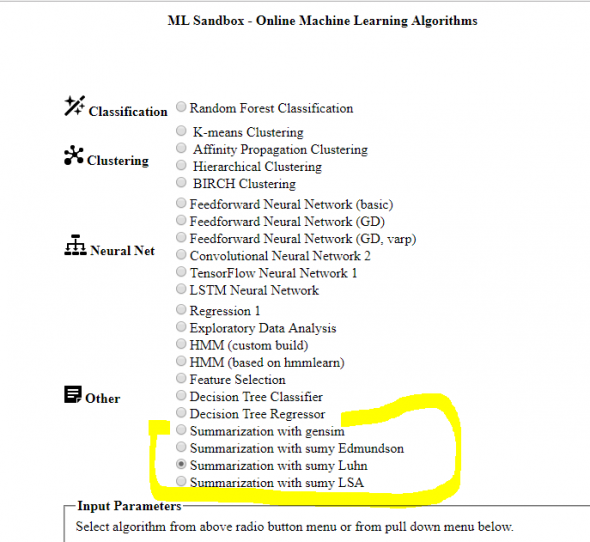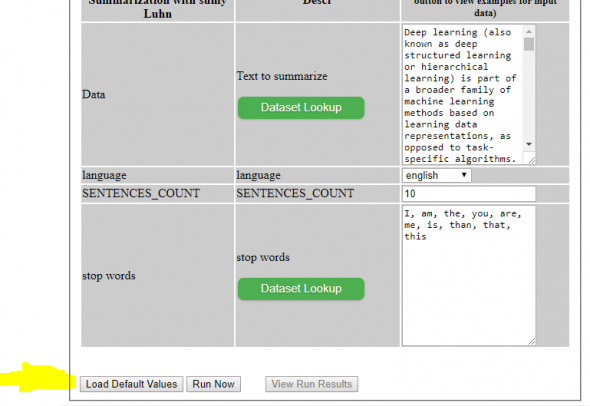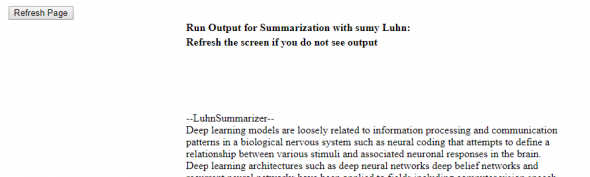## Automatic Text Summarization Online

In the previous post Automatic Text Summarization with Python I showed how to use different python libraries for text summarization. Recently I added text summarization modules to online site Online Machine Learning Algorithms. So now you can play with text summarization modules online and select best summary generator. This service is the free tool that allows to run some algorithms without coding or installing software modules.

Below are the steps how to use online text summarizer models of Machine Learning Algorithms tool.

## How to use online text summarizer algorithms

1. Access the link Online Machine Learning Algorithms : Online Machine Learning Algorithms tool.
Select text summarization algorithm that you want to run. There is one available with gensim and 3 with sumy python modules. We will use Luhn text summarizer algorithm. The algorithms from gensim and sumy python modules are still widely used in automatic text summarization which is part of the field of natural language processing.Running online text summarization step1

2. Input the data that you want to run or click on Load Default Values. Note that you need to enter about 10 sentences at least. It will not work if you enter just few words or just one sentence.Running online text summarization step2

3. Click Run now.

4. Click View Run Results link.Running online text summarization – example of output

5. Click Refresh Page button on this new page , you maybe will need click few times untill data output show up. Usually it takes less than 1 min, but it will depend how much data you need to process.
Scroll to the bottom page to see results.

If you try other text summarizers from this online tool you will see that there are some differences in generated text summaries.

## End Notes

In this post, we covered how to use online text summarizer models of Machine Learning Algorithms tool available here You can run online algorithms from gensim and sumy python modules.
Feel free to provide comments or suggestions.

## Automatic Text Summarization with Python

Automatic text summarization is the process of shortening a text document with software, in order to create a summary with the major points of the original document. The main idea of summarization is to find a subset of data which contains the “information” of the entire set. Such techniques are widely used in industry today. 

In this post we will review several methods of implementing text data summarization techniques with python. We will use different python libraries.

### Text Summarization with Gensim

1. Our first example is using gensim – well know python library for topic modeling. Below is the example with summarization.summarizer from gensim. This module provides functions for summarizing texts. Summarizing is based on ranks of text sentences using a variation of the TextRank algorithm. 

TextRank is a general purpose graph-based ranking algorithm for NLP. Essentially, it runs PageRank on a graph specially designed for a particular NLP task. For keyphrase extraction, it builds a graph using some set of text units as vertices. Edges are based on some measure of semantic or lexical similarity between the text unit vertices.

```
from gensim.summarization.summarizer import summarize
from gensim.summarization import keywords

import requests

# getting text document from Internet
text = requests.get('http://rare-technologies.com/the_matrix_synopsis.txt').text

# getting text document from file
fname="C:\\Users\\TextRank-master\\wikipedia_deep_learning.txt"
with open(fname, 'r') as myfile:
text=myfile.read()

#getting text document from web, below function based from 3
from bs4 import BeautifulSoup
from urllib.request import urlopen

def get_only_text(url):
"""
return the title and the text of the article
at the specified url
"""
page = urlopen(url)
soup = BeautifulSoup(page, "lxml")
text = ' '.join(map(lambda p: p.text, soup.find_all('p')))
return soup.title.text, text

print ('Summary:')
print (summarize(text, ratio=0.01))

print ('\nKeywords:')
print (keywords(text, ratio=0.01))

url="https://en.wikipedia.org/wiki/Deep_learning"
text = get_only_text(url)

print ('Summary:')
print (summarize(str(text), ratio=0.01))

print ('\nKeywords:')

# higher ratio => more keywords
print (keywords(str(text), ratio=0.01))
```

Here is the result for link https://en.wikipedia.org/wiki/Deep_learning
Summary:
In 2003, LSTM started to become competitive with traditional speech recognizers on certain tasks. Later it was combined with connectionist temporal classification (CTC) in stacks of LSTM RNNs. In 2015, Google\’s speech recognition reportedly experienced a dramatic performance jump of 49% through CTC-trained LSTM, which they made available through Google Voice Search. In the early 2000s, CNNs processed an estimated 10% to 20% of all the checks written in the US. In 2006, Hinton and Salakhutdinov showed how a many-layered feedforward neural network could be effectively pre-trained one layer at a time, treating each layer in turn as an unsupervised restricted Boltzmann machine, then fine-tuning it using supervised backpropagation. Deep learning is part of state-of-the-art systems in various disciplines, particularly computer vision and automatic speech recognition (ASR).

Keywords:
deep learning
learned
learn
learns
layer
layered
layers
models
model
modeling
images
image
recognition
data
networks
network
trained
training
train
trains

### Text Summarization using NLTK and Frequencies of Words

2. Our 2nd method is word frequency analysis provided on The Glowing Python blog . Below is the example how it can be used. Note that you need FrequencySummarizer code from  and put it in separate file in file named FrequencySummarizer.py in the same folder. The code is using NLTK library.

```
#note FrequencySummarizer is need to be copied from
# https://glowingpython.blogspot.com/2014/09/text-summarization-with-nltk.html
# and saved as FrequencySummarizer.py in the same folder that this
# script
from FrequencySummarizer import FrequencySummarizer

from bs4 import BeautifulSoup
from urllib.request import urlopen

def get_only_text(url):
"""
return the title and the text of the article
at the specified url
"""

page = urlopen(url)
soup = BeautifulSoup(page)
text = ' '.join(map(lambda p: p.text, soup.find_all('p')))

print ("=====================")
print (text)
print ("=====================")

return soup.title.text, text

url="https://en.wikipedia.org/wiki/Deep_learning"
text = get_only_text(url)

fs = FrequencySummarizer()
s = fs.summarize(str(text), 5)
print (s)
```

3. Here is the link to another example for building summarizer with python and NLTK.
This Summarizer is also based on frequency words – it creates frequency table of words – how many times each word appears in the text and assign score to each sentence depending on the words it contains and the frequency table.
The summary then built only with the sentences above a certain score threshold. 

### Automatic Summarization Using Different Methods from Sumy

4. Our next example is based on sumy python module. Module for automatic summarization of text documents and HTML pages. Simple library and command line utility for extracting summary from HTML pages or plain texts. The package also contains simple evaluation framework for text summaries. Implemented summarization methods:

Luhn – heurestic method
Edmundson heurestic method with previous statistic research
Latent Semantic Analysis
LexRank – Unsupervised approach inspired by algorithms PageRank and HITS
TextRank
SumBasic – Method that is often used as a baseline in the literature
KL-Sum – Method that greedily adds sentences to a summary so long as it decreases the KL Divergence. 

Below is the example how to use different summarizes. The usage most of them similar but for EdmundsonSummarizer we need also to enter bonus_words, stigma_words, null_words. Bonus_words are the words that we want to see in summary they are most informative and are significant words. Stigma words are unimportant words. We can use tf-idf value from information retrieval to get the list of key words.

```
from __future__ import absolute_import
from __future__ import division, print_function, unicode_literals

from sumy.parsers.html import HtmlParser
from sumy.parsers.plaintext import PlaintextParser
from sumy.nlp.tokenizers import Tokenizer
from sumy.summarizers.lsa import LsaSummarizer
from sumy.nlp.stemmers import Stemmer
from sumy.utils import get_stop_words

from sumy.summarizers.luhn import LuhnSummarizer
from sumy.summarizers.edmundson import EdmundsonSummarizer   #found this is the best as
# it is picking from beginning also while other skip

LANGUAGE = "english"
SENTENCES_COUNT = 10

if __name__ == "__main__":

url="https://en.wikipedia.org/wiki/Deep_learning"

parser = HtmlParser.from_url(url, Tokenizer(LANGUAGE))
# or for plain text files
# parser = PlaintextParser.from_file("document.txt", Tokenizer(LANGUAGE))

print ("--LsaSummarizer--")
summarizer = LsaSummarizer()
summarizer = LsaSummarizer(Stemmer(LANGUAGE))
summarizer.stop_words = get_stop_words(LANGUAGE)
for sentence in summarizer(parser.document, SENTENCES_COUNT):
print(sentence)

print ("--LuhnSummarizer--")
summarizer = LuhnSummarizer()
summarizer = LsaSummarizer(Stemmer(LANGUAGE))
summarizer.stop_words = ("I", "am", "the", "you", "are", "me", "is", "than", "that", "this",)
for sentence in summarizer(parser.document, SENTENCES_COUNT):
print(sentence)

print ("--EdmundsonSummarizer--")
summarizer = EdmundsonSummarizer()
words = ("deep", "learning", "neural" )
summarizer.bonus_words = words

words = ("another", "and", "some", "next",)
summarizer.stigma_words = words

words = ("another", "and", "some", "next",)
summarizer.null_words = words
for sentence in summarizer(parser.document, SENTENCES_COUNT):
print(sentence)
```

I hope you enjoyed this post review about automatic text summarization methods with python. If you have any tips or anything else to add, please leave a comment below.